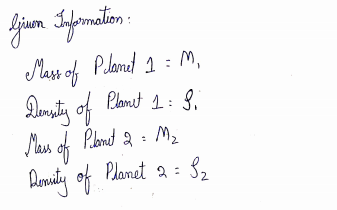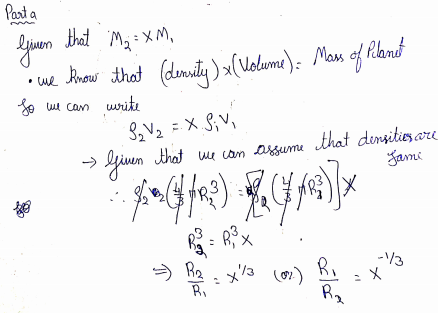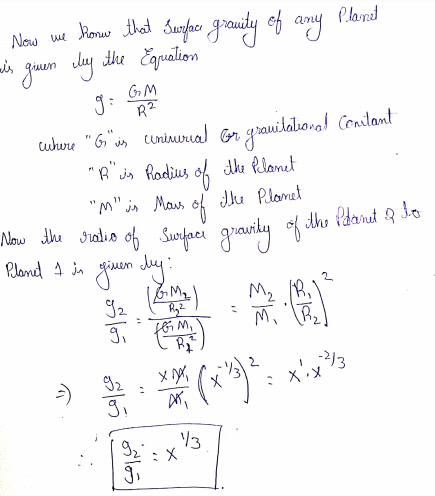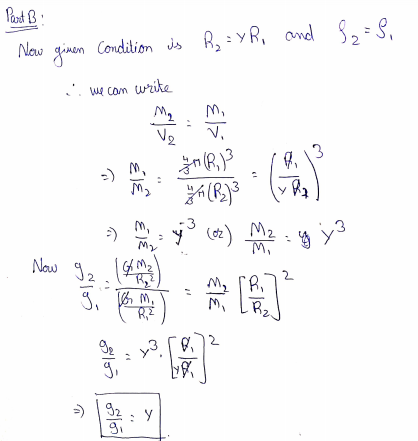# A planet has a mass of M1, a radius of R1, and a density of ρ1. A second planet has a mass of M2, a radius of R2, and a density of ρ2. This problem will explore the relationships between the surface gravities (g1 and g2) of the planets depending on the relative sizes of their masses, radii, and densities.a) Assume that planet 2 has X times the mass of planet 1, or M2 = XM1. The densities of both planets are the same. Write an expression for the ratio of the surface gravity of planet 2 to planet 1 in terms of X. b)Suppose now the radius of the second planet is Y times the size of the radius of the first planet, or R2 = YR1. Write an expression for the ratio of the surface gravities, g2/g1 in terms of Y assuming the densities are the same. c) Suppose now M2 = 8M1 and ρ2 = 8ρ1. What is the ratio of g2/g1 now (here we want the actual number; because you are writing a ratio, the number will be unitless)? d) Now suppose R2 = 10R1 and ρ2 = 10ρ1. Find the ratio of g2/g1 (again as a number and again the number will be unitless).

Question
4 views

A planet has a mass of M1, a radius of R1, and a density of ρ1. A second planet has a mass of M2, a radius of R2, and a density of ρ2. This problem will explore the relationships between the surface gravities (g1 and g2) of the planets depending on the relative sizes of their masses, radii, and densities.

a) Assume that planet 2 has X times the mass of planet 1, or M2 = XM1. The densities of both planets are the same. Write an expression for the ratio of the surface gravity of planet 2 to planet 1 in terms of X

b)Suppose now the radius of the second planet is Y times the size of the radius of the first planet, or R2 = YR1. Write an expression for the ratio of the surface gravities, g2/g1 in terms of Y assuming the densities are the same.

c) Suppose now M2 = 8M1 and ρ2 = 8ρ1. What is the ratio of g2/g1 now (here we want the actual number; because you are writing a ratio, the number will be unitless)?

d) Now suppose R2 = 10R1 and ρ2 = 10ρ1. Find the ratio of g2/g1 (again as a number and again the number will be unitless).

check_circle

Step 1Step 2Step 3...

### Want to see the full answer?

See Solution

#### Want to see this answer and more?

Solutions are written by subject experts who are available 24/7. Questions are typically answered within 1 hour.*

See Solution
*Response times may vary by subject and question.
Tagged in